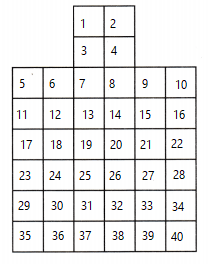# Texas Go Math Grade 3 Lesson 16.1 Answer Key Measure Area

Refer to our Texas Go Math Grade 3 Answer Key Pdf to score good marks in the exams. Test yourself by practicing the problems from Texas Go Math Grade 3 Lesson 16.1 Answer Key Measure Area.

## Texas Go Math Grade 3 Lesson 16.1 Answer Key Measure Area

Investigate

Area is the measure of the number of unit squares needed to cover a flat surface. A unit square is a square with a side length of 1 unit. It has an area of 1 square unit.Activity Materials; 1-inch grid paper; scissors

You can measure the area of the rectangles with 1-inch square tiles.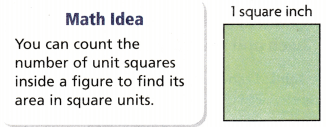Cut out eight 1-inch squares. Use the dashed lines as guides to place tiles for A-C.

A. Place 4 tiles on Rectangle A.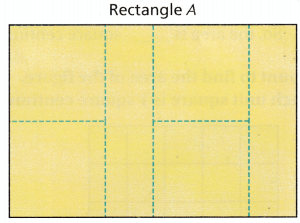Are there any gaps?_________
Are there any overlaps? _________
Jaime says that the area is 4 square inches.
Is Jaime’s measurement correct? _________
Are there any gaps? Yes
Are there any overlaps? No
Jaime says that the area is 4 square inches.
Is Jaime’s measurement correct? No

When you measure area, there can be no space, or gaps, between the tiles.

B. Place 8 tiles on Rectangle B.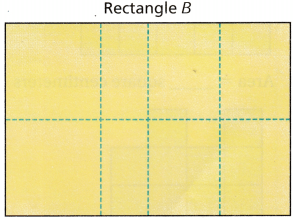Are there any gaps? _________
Are there any overlaps? _________
Jaime says that the area is 8 square inches. Is Jaime’s measurement correct? _________
Are there any gaps? Yes
Are there any overlaps? Yes
Jaime says that the area is 8 square inches. Is Jaime’s measurement correct? No
When you measure the area, the tiles cannot overlap.

C. Place 6 tiles on Rectangle C.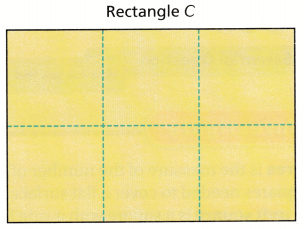Are there any gaps? _________
Are there any overlaps? _________
Jaime says that the area is 6 square inches. Is Jaime’s measurement correct? _________
So, the area of the rectangle is ___________ square inches.
Are there any gaps? No
Are there any overlaps? No
Jaime says that the area is 6 square inches. Is Jaime’s measurement correct? Yes
So, the area of the rectangle is  6 square inches.

Share and Show

Question 1.
Count to find the area of the large figure. Each unit square is 1 square centimeter.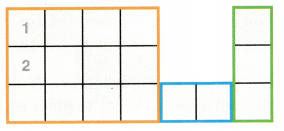Think: I can put the small shapes together if there are no gaps and no overlaps.
There are ________ unit squares in the figure.
So, the area is ________ square centimeters.Explanation:
There are 17 unit squares in the figure.
So, the area is 17 square centimeters.

Count to find the area of the figure. Each unit square is 1 square centimeter.

Question 2.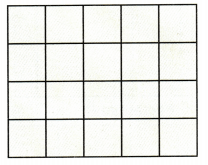Area = _________ square centimeters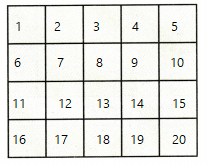Explanation:
Area = 20 square centimeters

Question 3.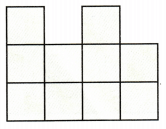Area = _________ square centimeters
Explanation: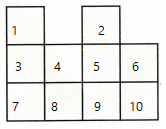Area = 10 square centimeters

Count to find the area of the figure. Each unit square is 1 square inch.

Question 4.Area = _________ square inchesExplanation:
Area = 6 square inches

Problem Solving

Question 5.
Danny is placing tiles on the floor of an office lobby. Each tile is 1 square meter. The diagram at the right shows the lobby. What is the area of the lobby?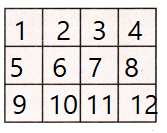Explanation:
Danny is placing tiles on the floor of an office lobby.
Each tile is 1 square meter.
The diagram at the right shows the lobby.
12 Square Meter is the area of the lobby

Question 6.
Multi-Step Angie is painting a space shuttle mural on a wall. Each section is one square foot. The diagram shows the unfinished mural. How many more square feet has Angie painted than NOT painted on her mural?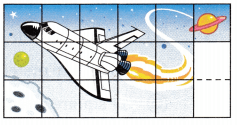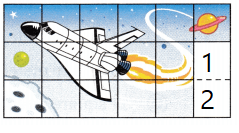Explanation:
Angie is painting a space shuttle mural on a wall.
Each section is one square foot.
The diagram shows the unfinished mural.
2 more square feet has Angie painted than NOT painted on her mural.

Question 7.
Write Math Sense or Nonsense? Tom places green square tiles on the figure at the right. He says that the figure has an area of 12 square units. Does his statement make sense? Explain.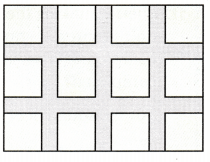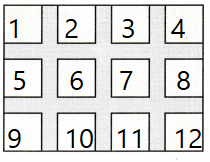Explanation:
Tom places green square tiles on the figure at the right.
He says that the figure has an area of 12 square units.
Yes, his statement make sense.

Question 8.
H.O.T. Pose a Problem Write an area problem that can be solved by using Rectangle A and Rectangle B. Then solve your problem.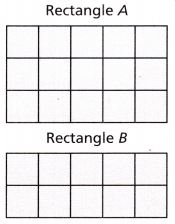Answer: What is the total area of Rectangle  A and Rectangle B
15 + 10 = 25
Explanation:Question 9.
H.O.T. Reasoning You measure the area of a table top with blue unit squares and green unit squares. Which unit square will give you a greater number of square units for area? Explain.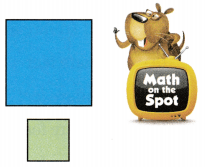Explanation:
When it is small the count will be more
when the square is big the count will become less.

Fill in the bubble for the correct answer choice.

Question 10.
Farmer Paul plants peas. The diagram shows his field. Each unit square is I square meter. What is the area of Farmer Paul’s field?(A) 28 square meters
(B) 24 square meters
(C) 30 square meters
(D) 11 square metersExplanation:
Farmer Paul plants peas.
The diagram shows his field.
Each unit square is I square meter.
24 is the area of Farmer Paul’s field.

Question 11.
Sheri is making the quilt shown in the diagram. Each unit square is 1 square foot. The blue squares are completed. What is the area of the quilt that Sheri has completed?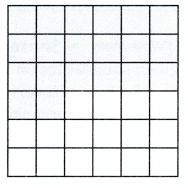(A) 36 square feet
(B) 17 square feet
(C) 20 square feet
(D) 16 square feetExplanation:
Sheri is making the quilt shown in the diagram.
Each unit square is 1 square foot.
The blue squares are completed.
20 is the area of the quilt that Sheri has completed

Question 12.
Multi-Step Use the diagram from Exercise 11. How many more square feet has Sheri completed than NOT completed for her quilt?
(A) 16 square feet
(B) 4 square feet
(C) 12 square feet
(D) 14 square feetExplanation:
16 more square feet has Sheri completed than NOT completed for her quilt.

Texas Test Prep

Question 13.
What is the area of the figure at the right? Each unit square is 1 square foot.(A) 3 square feet
(B) 15 square feet
(C) 13 square feet
(D) 10 square feet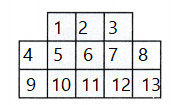Explanation:
The area of the figure is 13 square feet.

### Texas Go Math Grade 3 Lesson 16.1 Homework and Practice Answer Key

Count to find the area of the figure. Each unit square is 1 square centimeter.

Question 1.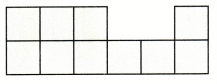Area = _________ square centimetersExplanation:
Area = 10 square centimeters

Question 2.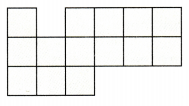Area = _________ square centimeters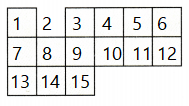Explanation:
Area = 15 square centimeters

Problem Solving

Question 3.
Kaylee is covering the top of a box with tiles. Each tile is 1 square centimeter. The diagram shows the top of the box. What is the area?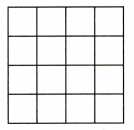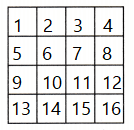Explanation:
Kaylee is covering the top of a box with tiles.
Each tile is 1 square centimeter.
The diagram shows the top of the box.
The area is 16 Square Centimeter

Question 4.
Miguel drew this puzzle. He divides the area into one-inch square sections. The diagram shows how much of the puzzle Miguel puts together. What is the area of the puzzle Miguel has completed so far?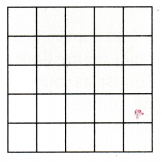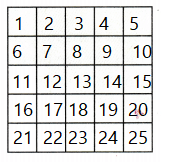Explanation:
Miguel drew this puzzle.
He divides the area into one-inch square sections.
The diagram shows how much of the puzzle Miguel puts together.
25 is the area of the puzzle Miguel has completed so far.

Question 5.
Dawn draws a picture that is 12 square inches. She draws another picture that is 12 square centimeters. Which picture is larger? Use centimeter and inch square paper to solve the problem. Explain how you solved the problem.
Answer: 12 inch is larger than 12 centimeter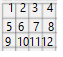Explanation:
12 inch is larger than the 12 centimeter

Lesson Check

Question 6.
Angelina is covering a closet floor with 1-foot square tiles. The green squares are completed. What area of the floor has Angelina covered?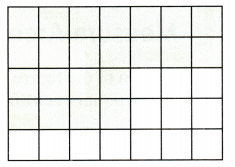(A) 22 square feet
(B) 35 square feet
(C) 24 square feet
(D) 20 square feetExplanation:
Angelina is covering a closet floor with 1-foot square tiles.
The green squares are completed.
35 Square feet area of the floor has Angelina covered

Question 7.
Multi-Step Dom is tiling a countertop. Each unit square is 1 square foot. The blue squares are completed. How many more square feet of countertop has Dom NOT completed, compared to what he has completed?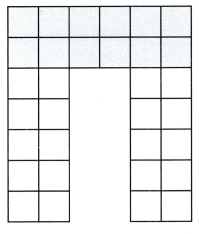(A) 12 square feet
(B) 20 square feet
(C) 32 square feet
(D) 8 square feet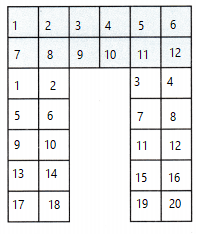20 – 12 = 8
Explanation:
Dom is tiling a countertop.
Each unit square is 1 square foot.
The blue squares are completed.
20 – 12 = 8
20 more square feet of countertop has Dom NOT completed, compared to what he has completed

Question 8.
Multi-Step A craft company uses 1-inch square tiles for the floor and closet of a dollhouse as shown in the diagram. An additional 7 square inches of tile will be used for a hallway. How many square inches of tile does the craft company need for the dollhouse?(A) 47 square inches
(B) 51 square inches
(C) 48 square inches
(D) 40 square inches